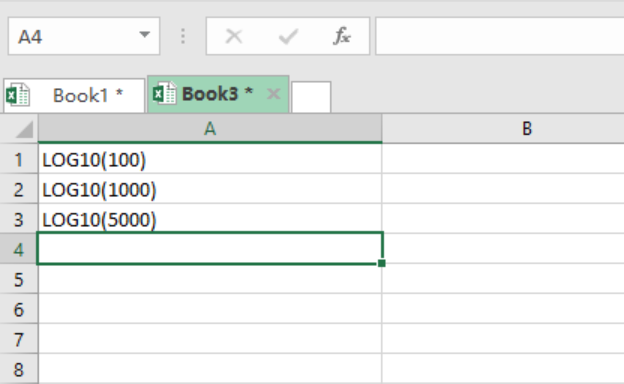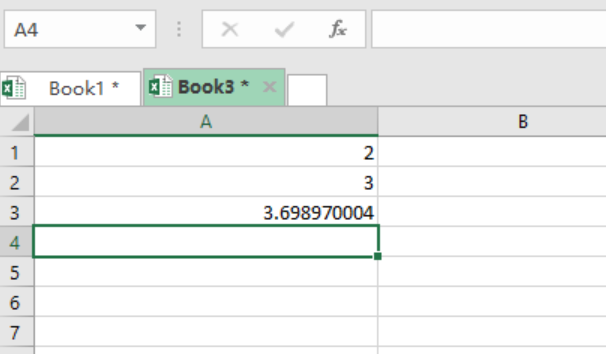Go Back

# How to use LOG10 function in Excel

We no longer have to worry about carrying out strenuous mathematical calculations that involve base 10 in excel. With the use of Excel Log10 function, we can be sure to get all the algorithm numbers that the formula is applied to. This article will explain the Log10 function step by step.

## Formula

`=LOG10 (number)`

## Arguments

Number- this specifies the number of which you want its logarithm.

## How the function is used

You need to first understand the parameters or arguments for the formula before you start using it. It is the parameters that will teach you on how to properly use the formula, thus knowing the formula alone isn’t much important.

## Parameters to use the Excel LOG10 function

1.       LOG10 function can be applied to all excel worksheet.
2.       LOG10 function returns a numeric value
3.       The number value in the application should be greater than 0

Note that this function only allows you to get the base 10 of positive numbers.

## ExampleFigure 1: Values for which we want to find logarithm for.

Procedure.

Insert the “Equals to” sign before the LOG10, and then Press Enter.Figure 2: Showing the LOG10 of the specified numbers.

## Notes

There are instances when one will get an error after using the LOG10 function. This can be due to the following two reasons:

#NUM! – This error will occur if you supply a negative or zero number.

#VALUE! – This error will occur if you supply a non-numeric number.

### Did this post not answer your question? Get a solution from connecting with the expert.

Another blog reader asked this question today on Excelchat: